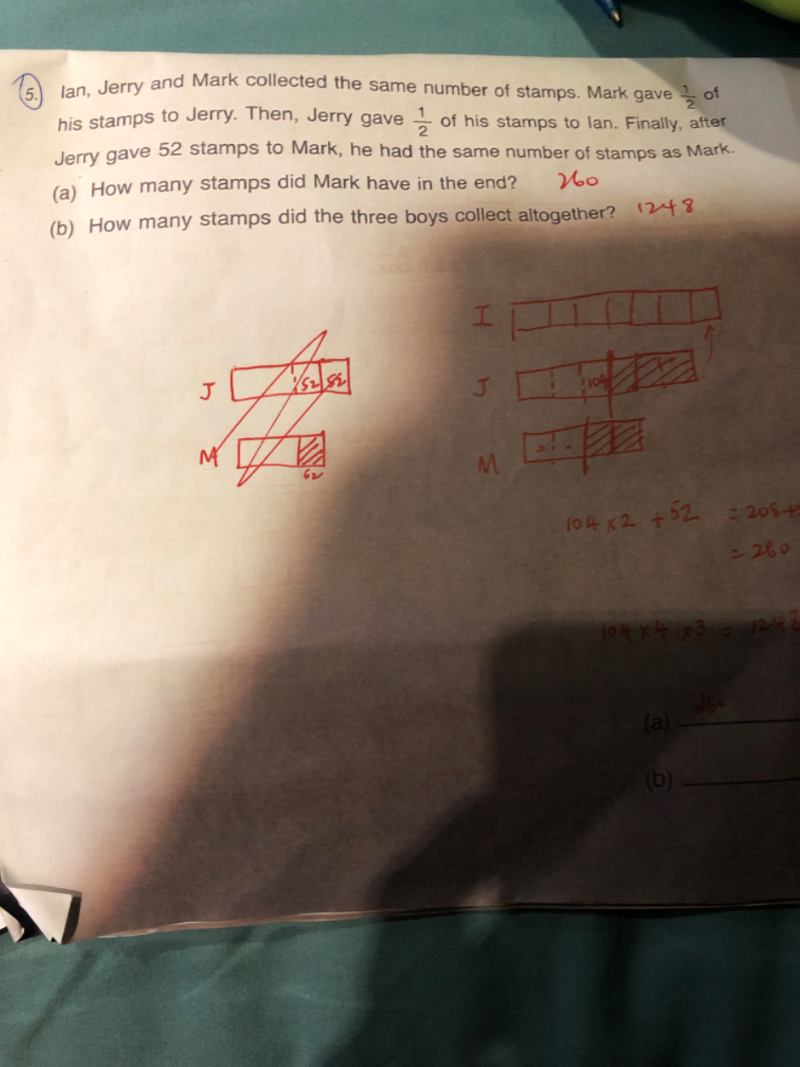# QuestionPls help

Ian                       Jerry                    Mark

At first                               u                            u                            u

M gave to J                                                   +(1/2)u               -(1/2)u

Update                              u                          (3/2)u                  (1/2)u

J gave I                  +(1/2)(3/2)u         -(1/2)(3/2)u

Update                         (7/4)u                     (3/4)u                  (1/2)u

J gave M                                                         -52                         +52

In the end                  (7/4)u                (3/4)u-52                (1/2)u+52

(a)  We know in the end, Jerry = Mark

So    (3/4)u-52   =  (1/2)u+52

(1/4) u   =  104

u        =  416

Hence in the end, Mark has  (416/2) + 52 = 260 stamps.

(b)   The boys started with 3u so

Stamps collected by the 3 boys altogether is 3×416 = 1248 stamps.

0 Replies 1 Like